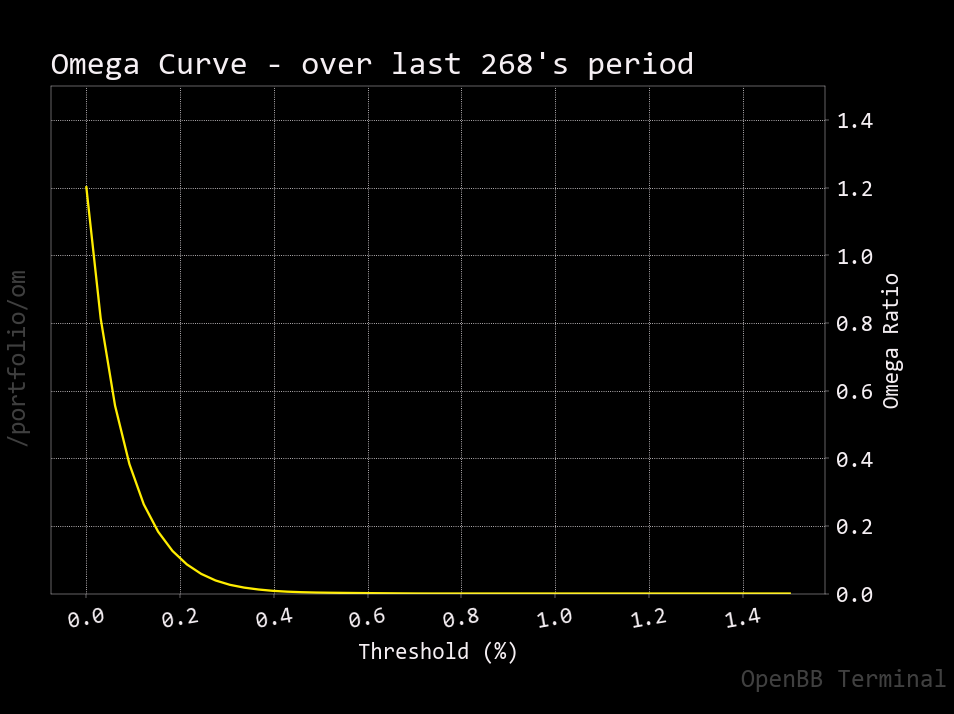# Om

``````usage: om [-s START] [-e END] [-h]
``````

Provides omega ratio of the selected portfolio.

The omega ratio is the probability weighted ratio of gains versus losses for a threshold return. This is in practice done by getting the sum of the returns above the threshold return and of the returns below it and then calculating the ratio between these sums. For more, read: https://en.wikipedia.org/wiki/Omega_ratio

``````optional arguments:
-s START, --start START
Start of the omega ratio threshold (default: 0)
-e END, --end END     End of the omega ratio threshold (default: 1.5)
-h, --help            show this help message (default: False)
``````